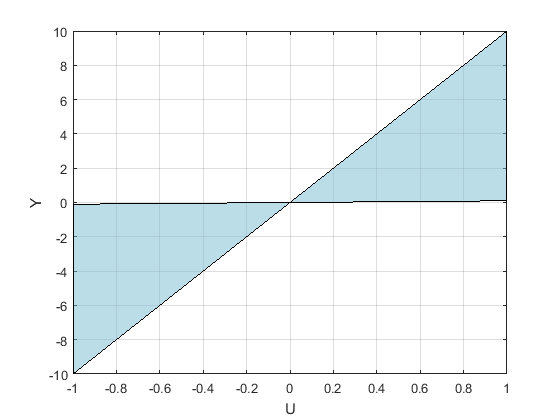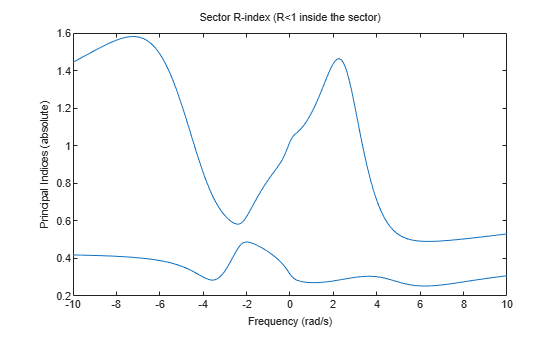# getSectorIndex

Compute conic-sector index of linear system

## Syntax

``RX = getSectorIndex(H,Q)``
``RX = getSectorIndex(H,Q,tol)``
``RX = getSectorIndex(H,Q,tol,fband)``
``````[RX,FX] = getSectorIndex(___)``````
``````[RX,FX,W1,W2,Z] = getSectorIndex(___)``````
``DX = getSectorIndex(H,Q,dQ)``
``DX = getSectorIndex(H,Q,dQ,tol)``

## Description

example

````RX = getSectorIndex(H,Q)` computes the relative index `RX` for the linear system `H` and the conic sector specified by `Q`. When `RX` < 1, all output trajectories y(t) = Hu(t) lie in the sector defined by:${\int }_{0}^{T}y{\left(t\right)}^{T}Q\text{\hspace{0.17em}}y\left(t\right)dt<0,$for all T ≥ 0. `getSectorIndex` can also check whether all I/O trajectories {u(t),y(t)} of a linear system G lie in the sector defined by:${\int }_{0}^{T}{\left(\begin{array}{c}y\left(t\right)\\ u\left(t\right)\end{array}\right)}^{T}Q\text{\hspace{0.17em}}\left(\begin{array}{c}y\left(t\right)\\ u\left(t\right)\end{array}\right)dt<0,$for all T ≥ 0. To do so, use `getSectorIndex` with ```H = [G;I]```, where `I = eyes(nu)`, and `nu` is the number of inputs of `G`.For more information about sector bounds and the relative index, see About Sector Bounds and Sector Indices.```
````RX = getSectorIndex(H,Q,tol)` computes the index with relative accuracy specified by `tol`.```
````RX = getSectorIndex(H,Q,tol,fband)` computes the passivity index by restricting the inequalities that define the index to a specified frequency interval. This syntax is available only when `Q` has as many negative eigenvalues as there are inputs in `H`. ```

example

``````[RX,FX] = getSectorIndex(___)``` also returns the frequency `FX` at which the index value `RX` is achieved. `FX` is set to `NaN` when the number of negative eigenvalues in `Q` differs from the number of inputs in `H`. You can use this syntax with any of the previous combinations of input arguments. ```
``````[RX,FX,W1,W2,Z] = getSectorIndex(___)``` also returns the decomposition of `Q` into its positive and negative parts, as well as the spectral factor `Z` when `Q` is dynamic. When `Q` is a matrix (constant sector bounds), `Z` = 1. You can use this syntax with any of the previous combinations of input arguments. ```
````DX = getSectorIndex(H,Q,dQ)` computes the index in the direction specified by the matrix `dQ`. If `DX` > 0, then the output trajectories of `H` fit in the conic sector specified by `Q`. For more information about the directional index, see About Sector Bounds and Sector Indices.The directional index is not available if `H` is a frequency-response data (`frd`) model.```
````DX = getSectorIndex(H,Q,dQ,tol)` computes the index with relative accuracy specified by `tol`.```

## Examples

collapse all

Test whether, on average, the I/O trajectories of $G\left(s\right)=\left(s+2\right)/\left(s+1\right)$ belong within the sector defined by:

`$S=\left\{\left(y,u\right):0.1{u}^{2}`

In U/Y space, this sector is the shaded region of the following diagram.The `Q` matrix corresponding to this sector is given by:

`$Q=\left[\begin{array}{cc}1& -\left(a+b\right)/2\\ -\left(a+b\right)/2& ab\end{array}\right];\phantom{\rule{1em}{0ex}}a=0.1,\phantom{\rule{0.2777777777777778em}{0ex}}b=10.$`

A trajectory $y\left(t\right)=G\phantom{\rule{0.1em}{0ex}}u\left(t\right)$ lies within the sector S when for all T > 0,

`$0.1{\int }_{0}^{T}u{\left(t\right)}^{2}<\phantom{\rule{0.2777777777777778em}{0ex}}\phantom{\rule{0.2777777777777778em}{0ex}}{\int }_{0}^{T}u\left(t\right)y\left(t\right)dt<\phantom{\rule{0.2777777777777778em}{0ex}}\phantom{\rule{0.2777777777777778em}{0ex}}10{\int }_{0}^{T}u{\left(t\right)}^{2}dt.$`

To check whether trajectories of `G` satisfy the sector bound, represented by `Q`, compute the R-index for `H = [G;1]`.

```G = tf([1 2],[1 1]); a = 0.1; b = 10; Q = [1 -(a+b)/2 ; -(a+b)/2 a*b]; R = getSectorIndex([G;1],Q)```
```R = 0.4074 ```

This resulting `R` is less than 1, indicating that the trajectories fit within the sector. The value of `R` tells you how much tightly the trajectories fit in the sector. This value, `R` = 0.41, means that the trajectories would fit in a narrower sector with a base 1/0.41 = 2.4 times smaller.

For systems with complex coefficients, `getSectorIndex` can return indices at a negative or a positive frequency depending on the `fband` you specify.

Load a state-space model with complex coefficients and complex sector matrix.

`load dataSysQ sys Q`

Compute the R-index and its frequency with a relative accuracy of 0.0001%. Also, specify `fband` = `[1,10]` to compute the index in the frequency interval [–10,–1] ∪ [1,10].

`[R,FX] = getSectorIndex(sys,Q,1e-6,[1,10])`
```R = 1.5811 ```
```FX = -7.2320 ```

In this interval, `sys` achieves an index of 1.5811 at a negative frequency value of –7.2320 rad/s. Use `sectorplot` to plot the indices in this range.

```opt = sectorplotoptions; opt.FreqScale = 'Linear'; opt.IndexScale = 'Linear'; w = linspace(-10,10,1000); sectorplot(sys,Q,w,opt)```Now compute the index in the frequency interval [–5,–1] ∪ [1,5]. To do so, specify `fband` = `[1,5]`.

`[r,f] = getSectorIndex(sys,Q,1e-6,[1,5])`
```r = 1.4630 ```
```f = 2.2499 ```

In this interval, `sys` achieves an index of 1.4630 at a positive frequency value of 2.2499 rad/s. Plot the indices in this range to confirm the result.

```w = linspace(-5,5,500); sectorplot(sys,Q,w,opt)```## Input Arguments

collapse all

Model to analyze against sector bounds, specified as a dynamic system model such as a `tf`, `ss`, or `genss` model. `H` can be continuous or discrete. If `H` is a generalized model with tunable or uncertain blocks, `getSectorIndex` analyzes the current, nominal value of `H`.

To analyze whether all I/O trajectories {u(t),y(t)} of a linear system G lie in a particular sector, use ```H = [G;I]```.

If `H` is a model array, then `getSectorIndex` returns the passivity index as an array of the same size, where:

`index(k) = getSectorIndex(H(:,:,k),___)`

Here, `index` is either `RX`, or `DX`, depending on which input arguments you use.

Sector geometry, specified as:

• A matrix, for constant sector geometry. `Q` is a symmetric square matrix that is `ny` on a side, where `ny` is the number of outputs of `H`.

• An LTI model, for frequency-dependent sector geometry. `Q` satisfies Q(s)’ = Q(–s). In other words, Q(s) evaluates to a Hermitian matrix at each frequency.

The matrix `Q` must be indefinite to describe a well-defined conic sector. An indefinite matrix has both positive and negative eigenvalues.

Relative accuracy for the calculated sector index. By default, the tolerance is 1%, meaning that the returned index is within 1% of the actual index.

Frequency interval for calculating the sector index, specified as an array of the form `[fmin,fmax]` with 0 ≤ `fmin` < `fmax`. When you provide `fband`, `getSectorIndex` restricts to the specified frequency interval the inequalities that define the index.

For models with complex coefficients, `getSectorIndex` computes the index in the range `[–fmax,–fmin]``[fmin,fmax]`. As a result, the function can return indices at a negative frequency.

Specify frequencies in units of `rad/TimeUnit`, where `TimeUnit` is the `TimeUnit` property of the dynamic system model `H`.

Direction in which to compute directional sector index, specified as a nonnegative definite matrix. The matrix `dQ` is a symmetric square matrix that is `ny` on a side, where `ny` is the number of outputs of `H`.

## Output Arguments

collapse all

Relative index of the system `H` for the sector specified by `Q`, returned as a scalar value, or an array if `H` is an array. If `RX` < 1, then the output trajectories of `H` fit inside the cone of `Q`.

The value of `RX` provides a measure of how tightly the output trajectories of `H` fit inside the cone. Let the following be an orthogonal decomposition of the symmetric matrix `Q` into its positive and negative parts.

`$Q={W}_{1}{W}_{1}^{T}-{W}_{2}{W}_{2}^{T},\text{ }{W}_{1}^{T}{W}_{2}=0.$`

(Such a decomposition is readily obtained from the Schur decomposition of `Q`.) Then, `RX` is the smallest R that satisfies:

`${\int }_{0}^{T}y{\left(t\right)}^{T}\left({W}_{1}{W}_{1}^{T}-{R}^{2}{W}_{2}{W}_{2}^{T}\right)\text{\hspace{0.17em}}y\left(t\right)dt<0,$`

for all T ≥ 0. Varying R is equivalent to adjusting the slant angle of the cone specified by Q until the cone fits tightly around the output trajectories of `H`. The cone base-to-height ratio is proportional to R.

Frequency at which the index `RX` is achieved, returned as a scalar, or an array if `H` is an array. In general, the index varies with frequency (see `sectorplot`). The returned value is the largest value over all frequencies. `FX` is the frequency at which this value occurs, returned in units of `rad/TimeUnit`, where `TimeUnit` is the `TimeUnit` property of `H`.

`FX` can be negative for systems with complex coefficients.

Positive and negative factors of `Q`, returned as matrices. For a constant `Q`, `W1` and `W2` satisfy:

`$Q={W}_{1}{W}_{1}^{T}-{W}_{2}{W}_{2}^{T},\text{ }{W}_{1}^{T}{W}_{2}=0.$`

Bistable model in the factorization of `Q`, returned as:

• If `Q` is a constant matrix, `Z` = 1.

• If `Q` is frequency-dependent, then `Z` is a state-space (`ss`) model such that:

`$Q\left(j\omega \right)=Z{\left(j\omega \right)}^{H}\left({W}_{1}{W}_{1}^{T}-{W}_{2}{W}_{2}^{T}\right)Z\left(j\omega \right).$`

Directional sector index of the system `H` for the sector specified by `Q` in the direction `dQ`, returned as a scalar value, or an array if `H` is an array. The directional index is the largest τ which satisfies:

`${\int }_{0}^{T}y{\left(t\right)}^{T}\left(Q+\tau dQ\right)\text{\hspace{0.17em}}y\left(t\right)dt<0,$`

for all T ≥ 0.